Ch 2. Particle Force and Acceleration Multimedia Engineering Dynamics Rect.Coord. Normal/Tang. Coord. PolarCoord. Orbital Mechanics Computational Mechanics
 Chapter - Particle - 1. General Motion 2. Force & Accel. 3. Energy 4. Momentum - Rigid Body - 5. General Motion 6. Force & Accel. 7. Energy 8. Momentum 9. 3-D Motion 10. Vibrations Appendix Basic Math Units Basic Equations Sections Search eBooks Dynamics Fluids Math Mechanics Statics Thermodynamics Author(s): Kurt Gramoll ©Kurt GramollDYNAMICS - CASE STUDY SOLUTION Solution of a)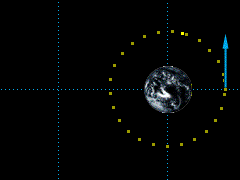Circular Orbit, ε = 0 The initial velocity required to give the satellite a circular orbit can be found from,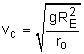where   g = 32.2 ft/s2             RE = 3.960 mi = 20.9 x 106 ft             ro = 10,000 mi = 52.8 x 106 ft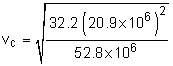= 16,300 ft/s Solution of b)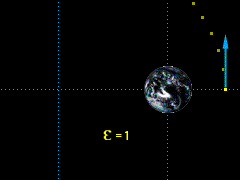Parabolic Orbit, ε =1 The minimum initial velocity required to escape the earth's gravitational pull can be found from: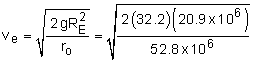= 23.1 x 103 ft/s Solution of c)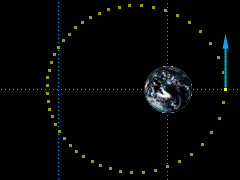Elliptical Orbit, 0 < ε < 1 To find the maximum radius, the eccentricity of the orbit must be determined when the initial velocity of the satellite is 20,000 ft/sec.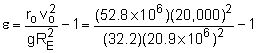= 0.500 Because 0 < ε < 1, the orbit must be elliptical, and thus the maximum radius occurs at apogee.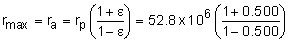= 158 x 106 ft = 29,900 miles

Practice Homework and Test problems now available in the 'Eng Dynamics' mobile app
Includes over 400 problems with complete detailed solutions.
Available now at the Google Play Store and Apple App Store.